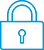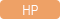cancel
Showing results for
Did you mean:It has been a while since anyone has replied. Simply ask a new question if you would like to start the discussion again.Level 2
16 9 0 0
Message 1 of 3
542
Flag Post

# HP Prime will not calculate slope in graphing from function defined by programHP Recommended
HP Prime

My Prime calculates correctly, on the graphing page, the slope of a graphed function whose formula is typed into the Symb page. It will also graph from a function defined in the Program section, and even calculate the area under the graph. However, it will not return a value for the slope of such a function (graph), or solve for a root or extremum (for programs of more than one line), even though those operations work correctly for functions defined by formulas typed into Symb. Is there a way around this? If not, does anyone understand why it works this way?

Tags (1)
2 REPLIES 2Level 8
747 744 121 187
Message 2 of 3
Flag PostHP Recommended

Example?

How would the derivative or integral of your "program" be found?

TW

Although I work for the HP calculator group as a head developer of the HP Prime, the views and opinions I post here are my own.Level 2
16 9 0 0
Message 3 of 3
Flag PostHP Recommended

Tim Wessman,

I graph a function defined in a program in the same way I graph a function whose formula is typed into Symb in the Prime except that instead of putting the formula, such as "x^2-1", into Symb, I put in the program name, such as "FLTR(X)" (with "X" as the variable name), which program has been defined in the Program section of the Prime. Then, I find the derivative at a point or the integral between specified limits of FLTR(X) in the same way I would for the typed-in function X^2-1, except that it works for the integral, but doesn't for the derivative (if the programs has more than one line).

† The opinions expressed above are the personal opinions of the authors, not of HP. By using this site, you accept the Terms of Use and Rules of Participation